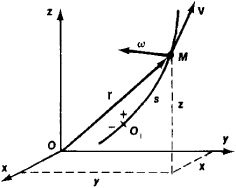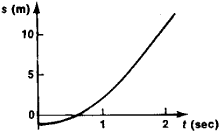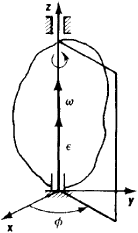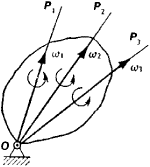# kinematics

(redirected from kinematic)
Also found in: Dictionary, Thesaurus, Medical, Wikipedia.
Related to kinematic: kinematic viscosity

## kinematics:

see dynamicsdynamics,
branch of mechanics that deals with the motion of objects; it may be further divided into kinematics, the study of motion without regard to the forces producing it, and kinetics, the study of the forces that produce or change motion.
.

## Kinematics

That branch of mechanics which deals with the motion of a system of material particles without reference to the forces which act on the system. Kinematics differs from dynamics in that the latter takes these forces into account. See Dynamics

For a single particle moving in a straight line (rectilinear motion), the motion is prescribed when the position of the particle is known as a function of the time. Plane kinematics of a particle is concerned with the specification of the position of a particle moving in a plane by means of two independent variables. The kinematics of a particle in space is concerned with the ways in which three independent coordinates may be chosen to specify the position of the particle at a given time, and with the relations between the first and second time derivatives of these coordinates and the components of velocity and acceleration of the particle.

Among the coordinate systems studied in kinematics are those used by observers who are in relative motion. In nonrelativistic kinematics the time coordinate for each such observer is assumed to be the same, but in relativistic kinematics proper account must be taken of the fact that lengths and time intervals appear different to observers moving relative to each other. See Relativity

## Kinematics

a branch of mechanics dealing with the study of geometric properties of the motion of bodies, without taking into account their masses or forces acting on them. The discussion that follows refers to the kinematics of motions treated in classical mechanics (motions of macroscopic bodies at velocities that are low in comparison with speed of light).

The methods and relationships established in kinematics are applied in the kinematic study of motions, particularly in calculations of the transmission of motion in various mechanisms and machines, as well as in solving problems of dynamics. Kinematics is subdivided into kinematics of particles, kinematics of rigid bodies, and kinematics of a continuous variable medium (a deformable body, a fluid, or a gas), depending on the properties of the object being studied.

In kinematics the motion of any object is studied with reference to some other body (a reference body), with which a frame of reference is associated (the axes x, y, and z in Figure 1). The frame of reference is used to define the position of the moving body relative to the reference body at various moments. The choice of a frame of reference is arbitrary and depends on the purpose of the research. For example, in studying the motion of a wheel of a railroad car relative to the rail the frame of reference is associated with the ground. In studying the motion of the same wheel relative to the body of the car, the frame of reference is associated with the body. The motion of the object under study is considered to be specified (known) if certain equations—the equations of motion (or graphs or tables)—are known; such equations define the position of the object relative to the frame of reference at any moment. The main problem in kinematics is the determination (with the aid of a particular mathematical method) of a means of specifying the motion of points or bodies and the determination from their equations of motion of the corresponding kinematic characteristics, such as trajectory, velocity, and acceleration of moving points and angular velocity and angular acceleration of rotating bodies. Three methods (the natural, coordinate, and vector methods) are used to specify the motion of a point.Figure 1

The natural (or trajectory) method is used if the trajectory of a point relative to the chosen frame of reference is known. The position of the point is determined by the distance s = O1M from the chosen point of origin O1 on the trajectory; the distance is measured along the arc of the trajectory and is used with the appropriate sign (see Figure 1). The law of motion is given by the equation s = f(t) which describes the dependence of s on time t. For example, if s = 3t2 – 1, then at the initial moment t0 = 0, s0 = – 1 m (a point located 1 m to the left of the origin O1). At t1 = 1 sec, s1 = 2m (a point 2 m to the right of O1), and so on. The dependence of s on t can also be represented by a motion diagram, in which time is plotted in a chosen scale along the t axis and distance is plotted along the s axis (see Figure 2), or by a table, where the values of t are given in one column and the corresponding values of s in another column (this method is used in railroad schedules).Figure 2

In the coordinate method, the position of a point relative to the frame of reference is defined by any three coordinates—for example, by the rectangular Cartesian coordinates x, y, and z. The law of motion is given by three equations x = f1(t), y = f2(t) and z = f3(t). The trajectory of a point may be found by eliminating the time t from these equations.

In the vector method, the position of a point relative to the frame of reference is determined by the radius vector r, drawn from the point of origin to the moving point. The law of motion is given by the vector equation r = r (t). The trajectory of the point is the hodograph of the vector r.

The basic kinematic characteristics of a moving point are its velocity and acceleration, the values of which are determined by equations of motion, using first and second derivatives with respect to time of s, of x, y, and z, or of r.

The methods for specifying the motion of a rigid body depend on the type of its motion. The number of equations of motion depends on the number of degrees of freedom of the body. The simplest motions of a rigid body are translation and rotation. In translation all points of a body move identically; its motion is formulated and studied in the same way as the motion of one point. In rotation around a fixed axis z (Figure 3), the body has one degree of freedom; its position is determined by the angle of rotation ϕ, and the law of motion is given by the equation ϕ = f(t). The basic kinematic characteristics of the body are its angular velocity ѡ = dϕ/dt and angular acceleration = dѡ/dt The quantities ѡ and are depicted as vectors directed along the axis of rotation. If ѡ and ѡ are known, the velocity and acceleration of any point of the body may be determined.Figure 3

The motion of a body having only one fixed point and three degrees of freedom—for example, a gyroscope or a top—is more complex. In this case a position of the body relative to the frame of reference is determined by any three angles (for example, the Eulerian angles: precession, nutation, and spin). The law of motion is determined by equations that describe the dependence of the angles on time. The basic kinematic characteristics of a body are the instantaneous angular velocity ѡ and the instantaneous angular acceleration ɛ. The motion of the body involves a series of elementary turns around instantaneous rotational axes OP, which pass through the fixed point O (Figure 4) and continuously change their direction.Figure 4

The most general case is that of the motion of a free rigid body having six degrees of freedom. The position of the body is determined by three coordinates of one of its points, called the pole (in problems of dynamics the center of gravity is assumed to be the pole), and by three angles chosen in the same way as for a body with a fixed point. The law of motion for the body is given by six equations that describe the dependence of the coordinates and angles on time. The motion of the body involves translation along the pole and a rotation around the pole, in the same way as around a fixed point. Examples of such motion are the motion of an artillery projectile, the motion of an airplane engaged in aerobatics, and the motion of heavenly bodies. The basic kinematic characteristics of a body are its velocity and acceleration of the translational part of the motion, which are equal to the velocity and acceleration of the pole, and angular velocity and acceleration of the rotation of the body around the pole. All these characteristics, like the kinematic characteristics for a body with a fixed point, are computed from the equations of motion; if the characteristics are known, the velocity and acceleration of any point of the body may be determined. A special case of the motion being considered is the plane motion of a rigid body, in which all of its points move parallel to a plane. Such motion is performed by components of many mechanisms and machines.

The compound motion of points and bodies—that is, motion examined simultaneously with respect to two or more frames of reference that are moving relative to each other—is also studied in kinematics. In this case one of the frames of reference is regarded as the system of absolute coordinates (also called the conventionally fixed system), and the frame of reference moving with respect to the primary system is called the system of relative coordinates. In the general case there may be several systems of relative coordinates.

In studying compound motion of a point, its motion—as well as its velocity and acceleration—is called conventionally absolute with respect to the system of absolute coordinates and conventionally relative with respect to the system of relative coordinates. The motion of the system of relative coordinates itself and of all points of space physically connected to it is called the motion of transport, and the velocity and acceleration of the point of the system of relative coordinates with which the moving point coincides at a given moment are called the velocity and acceleration of following. For example, if the system of absolute coordinates is connected with a shore and the system of relative coordinates is connected with a steamship moving on a river, and if the rolling of a ball on the deck of the ship is considered (the ball is taken to be a point), then the velocity and acceleration of the ball with respect to the deck are relative, whereas those with respect to the shore are absolute. However, the velocity and acceleration of a point of the deck touched by the ball at a given moment are the transport characteristics for the ball. Analogous terminology is used in studying compound motion of rigid bodies.

The main problems in kinematics of compound motions are the determination of the relations between the kinematic characteristics of the absolute and relative motions of a point (or a body) and the motion characteristics of the system of relative coordinates (that is, the motion of transport). For a point the corresponding equations are as follows: the absolute velocity of a point is equal to the vector sum of the relative velocity and the transport velocity,

Vabs = Vrel + Vtr

and the absolute acceleration of a point is equal to the vector sum of three accelerations (the relative, transport, and rotational, or Coriolis),

Wabs = Wrel + Wtr + Wcor

For a rigid body, when all the component motions (that is, the relative motion and the motion of transport) are translational the absolute motion is also translational, with a velocity equal to the sum of the velocities of the component motions. If the component motions of a body are those of rotation around axes intersecting at one point (as in a gyroscope), then the resultant motion will also be rotation around the point, with an instantaneous angular velocity equal to the vector sum of the angular velocities of the component motions. If the component motions of a body are both translational and rotational, then the resultant motion will usually consist of a series of instantaneous helical motions.

The kinematics of a continuous medium establishes methods for formulating a specifying the motion of such a medium, studies the general theory of deformation, and gives the continuity equations, which reflect the conditions for continuity in a medium.

S. M. TARG

## kinematics

(mechanics)
The study of the motion of a system of material particles without reference to the forces which act on the system.

## kinematics

the study of the motion of bodies without reference to mass or force
References in periodicals archive ?
This study utilised a test-retest design to determine intersession reliability of both short sprint performance and the kinematic variables, where each change score was measured in respect to each individual over two sessions (Hopkins, 2008).
Interestingly, there were no common kinematic variables that were significantly correlated to accuracy between the two different body orientations.
The aim of this research is to develop an algorithm kinetic research of velocities of the points that coincide with geometric centers kinematic pairs of structure group of the 4th class and 3rd order with movable close-cycle formed by connecting rod and two complex links.
However, hybrid kinematic structures, consisting or rotary and translations joints, are being realized in work cell configurations, which introduces unique challenges for path planning, and obstacle avoidance was well as the robots' capabilities for a wide range of product / process related tasks.
The primary goal of this study was to validate the kinematic responses of the THOR MB and FE models with the responses of the physical THOR dummy in rollover experiments presented previously .
Normally, for vertical feed kinematic chains, in case of enclosures, slides and even cross beams, if operating axes of CNC machine tools are involved , balancing systems with reducing valves, pressure regulating pumps or closed loop systems with accumulators are used .
Kinematic and kinetic analyses allow us to evaluate this "avoidance behavior" under realistic loading conditions.
Thus, when we modify the assembly model during analysis-redesign-reanalysis cycles, the kinematic functions can be directly validated.
Kinematic parameter analysis of the rocket lifting mechanism indicates that the mechanism ensures effective transition of the rocket from transportation to the launching mode.
Normally kinematic analysis is performed prior to detailed investigations in almost all slope stability analysis(Kulatilake et al.
The kinematic viscosity is proportional to the flow time:
The EXCM Mini H Handler's parallel kinematic system is extremely energy efficient compared to traditional Cartesian positioning systems in which one motor must transport the weight of the other.

Site: Follow: Share:
Open / Close